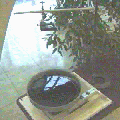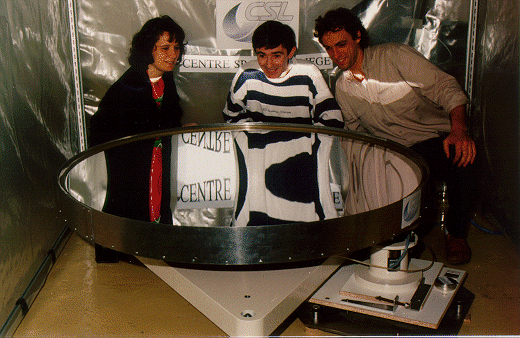The LMT Didactical experiment

J. Surdej and A. Pospieszalska-Surdej

What is a liquid mirror?

As we all know, a perfect reflecting parabola represents the ideal contour for a mirror to focus parallel rays of light into a single point.

It is therefore of the greatest importance to have realized that a rotating liquid surface takes the shape of a parabola under the constant pull of gravity and a centrifugal acceleration, which grows stronger as the distance from the central axis increases (E. Cappoci, 1850). The parabolic shape occurs because a liquid surface always sets it local surface perpendicular to the net acceleration it experiences, which in this case becomes stronger and less inclined with distance from the central axis (see Figure 1).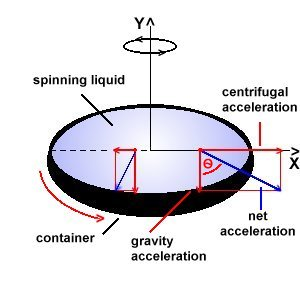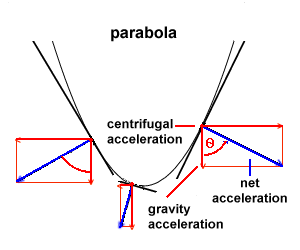Figure 1: Acting forces on a liquid set in a rotating container Figure 2: Parabolic shape of the spinning liquid with the distribution of forces acting on a single liquid particle

Indeed, inspection of the above figure clearly shows that the tangent dy/dx to the liquid surface may be expressed as (see Figure 2)

dy/dx = tg() =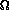2x / g, (1)

where2x and g represent the centrifugal force and gravity acting on a mass unit (see the red dot in Figure 1and Figure 2). Integration of the above differential equation leads to the solution

y =2x2/(2g), (2)

which, by analogy with the equation of a parabola caracterized by the focal length f, i.e.

y = x2/(4f),       (3)

implies that the focal length of the liquid mirror is merely given by the relation

f = g/(22).      (4)

A focal length of approximately 40 cm is obtained for a value of the angular velocity of 33 turns per minute.